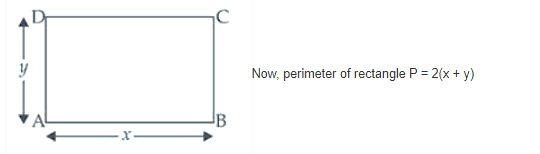# Find the dimensions of the rectangle of perimeter 36 cm`
Question:

Find the dimensions of the rectangle of perimeter 36 cm which will sweep out a volume as large as possible, when revolved about one of its sides. Also find the maximum volume.

Solution:

Let’s consider x and y to be the length and breadth of given rectangle ABCD.

According to the question, the rectangle will be resolved about side AD which making a cylinder with radius x and height y.

So, the volume of the cylinder V = πr2h = πx2y …. (1)36 = 2(x + y)

18 = x + y

y = 18 – x ….. (ii)

Putting the value of y in the equation (i), we get

V = πx2(18 – x) = π(18x2 – x3)

Differentiating both sides w.r.t. x, we get

dV/dx = π(36x – 3x2) …. (iii)

For local maxima and local minima dV/dx = 0

π(36x – 3x2) = 0

36x – 3x2 = 0

3x(12 – x) = 0

x ≠ 0 and 12 – x = 0 ⇒ x = 12

From equation (ii), we have

y = 18 – 12 = 6

Differentiating equation (iii) w.r.t. x, we get

d2V/dx2 = π(36 – 6x)

At x = 12,

d2V/dx2 = π(36 – 6 x 12) = π(36 – 72)

= -36 π < 0 maxima

Now, volume of the cylinder so formed = πx2y

= π x (12)x (6) = π(144)2 x 6 = 864π cm3

Therefore, the required dimension are 12 cm and 6 cm and the maximum volume is 864π cm3.